# NCERT Solutions For Class 10 Maths Chapter 7 Exercise 7.4

## Chapter 7 Ex.7.4 Question 1

Determine the ratio in which the line \begin{align}2x + y - 4 = 0\end{align} divides the line segment joining the points \begin{align} A(2, -2)\end{align} and \begin{align}B(3,7)\end{align}.

### Solution

Reasoning:

The coordinates of the point $$P(x, y)$$ which divides the line segment joining the points $${A(x_1, y_1) \;\text{and}\; B(x_2, y_2)}$$, internally, in the ratio $${m_1:m_2}$$ is given by the Section Formula.

\begin{align}P(x,y) \! = \! \left[ \frac{m x_2 \! + \! n x_1}{m \! + \! n},\frac{m y_2 \! + \! n y_1}{m \! + \! n} \right] \end{align}

What is known?

The $$x$$ and $$y$$ co-ordinates of the points $$A$$ and $$B$$.

The equation of the straight line i.e. \begin{align}2x + y - 4 = 0\end{align}

What is unknown?

The ratio in which the line \begin{align}2x + y - 4 = 0\end{align} divides the line segment joining the points \begin{align}A(2,-2)\;\text{ and }\; B(3,7)\end{align}

Steps:

From the Figure,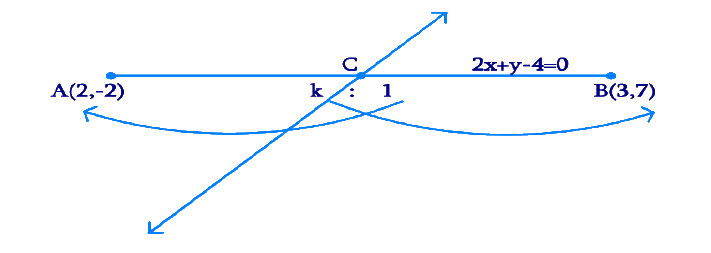Given,

• Let the given line \begin{align}2x + y - 4 = 0\end{align} divide the line segment joining the points \begin{align}A(2,- 2)\;\text{ and }\;B(,7)\end{align} in a ratio $$k : 1$$ at point $$C$$.

Coordinates of the point of division,

\begin{align}C(x,y) = \left[ {\frac{{3k + 2}}{{k + 1}},\;\frac{{7k - 2}}{{k + 1}}} \right]\end{align}

This point $$C$$ also lies on

$$2x + y - 4 = 0 \;\; \ldots(1)$$

By substituting the values of \begin{align}C(x,y)\end{align} in Equation $$(1)$$,

Therefore,

\begin{align}2\left[ {\frac{{3k + 2}}{{k + 1}}} \right] \!\!+ \!\! \left[ {\frac{{7k - 2}}{{k + 1}}} \right] \! - \! 4 \! = \! 0 \end{align}

\begin{align} \left[\frac{{6k \! + \! 4 \! + \! 7k \! - \! 2 \! - \! 4k \! - \! 4}}{{k \! + \! 1}}\right] \! = \! 0\end{align}

(By Cross multiplying & transposing)

\begin{align} 9k - 2 & = 0 \\k & = \frac{2}{9}\end{align}

Therefore, the ratio in which the line \begin{align}2x + y - 4 = 0\end{align} divides the line segment joining the points \begin{align}A(2,- 2) \; \text{ and }\;B(3,7)\end{align} is $$2:9$$ internally.

## Chapter 7 Ex.7.4 Question 2

Find a relation between $$x$$ and $$y$$ if the points \begin{align}(x,y), (1,2)\end{align} and \begin{align}(7,0)\end{align} are collinear.

### Solution

Reasoning:

Three or more points are said to be collinear if they lie on a single straight line .

What is known?

The $$x$$ and $$y$$ co-ordinates of the points.

What is unknown?

The relation between $$x$$ and $$y$$ if the points \begin{align}(x,y), (1,2)\end{align} and \begin{align}(7,0)\end{align} are collinear.

Steps:

Given,

• \begin{align}\text{Let } A(x_1,y_1) =(x,y)\end{align}
• \begin{align}\text{Let } B(x_2,y_2) =(1,2)\end{align}
• \begin{align}\text{Let } C(x_3,y_3) =(7,0)\end{align}

If the given points are collinear, then the area of triangle formed by these points will be $$0$$.

Area of a triangle

\begin{align}=\frac{1}{2} \begin{bmatrix} x_1 \left( y_2 - y_3 \right) + \\ x_2 \left( y_3 - y_1 \right) + \\ x_3 \left( y_1 - y_2 \right) \end{bmatrix} \;\;\dots(1) \end{align}

By substituting the values of vertices, $$A$$, $$B$$, $$C$$ in the Equation $$(1)$$,

Area of a triangle

\begin{align} & \! = \! \frac{1}{2}\begin{bmatrix} x(2 \! - \! 0) \! + \! 1(0 \! - \! y) \! +\\ 7(y \! - \! 2) \end{bmatrix} \\&0 \! = \! \frac{1}{2} \begin{bmatrix} 2x \! - \! y \! + \! 7y \! - \! 14 \end{bmatrix} \\0 & \! = \! \frac{1}{2}\left(2x \! + \! 6y \! - \! 14 \right)\\2x \! + \! 6y \! - \! 14 & \! = \! 0\\ x \! + \! 3 y \! - \! 7 & \! = \! 0\end{align}

This is the required relation between $$x$$ and $$y$$.

## Chapter 7 Ex.7.4 Question 3

Find the centre of a circle passing through the points

$$\left( {6,- 6} \right), \left( {3, - 7} \right){\text{ and }} \left( {3,3} \right).$$

### Solution

Reasoning:

The distance between the two points can be measured using the Distance Formula which is given by:

Distance Formula

$$\text=\sqrt{\left(x_{1}-x_{2}\right)^{2}+\left(y_{1}-y_{2}\right)^{2}}$$

What is known?

The $$x$$ and $$y$$ co-ordinates of the points.

What is unknown?

The centre of the circle passing through the points

$$\left( {6,- 6} \right), \left( {3, - 7} \right){\text{ and }} \left( {3,3} \right).$$

Steps:

From the Figure,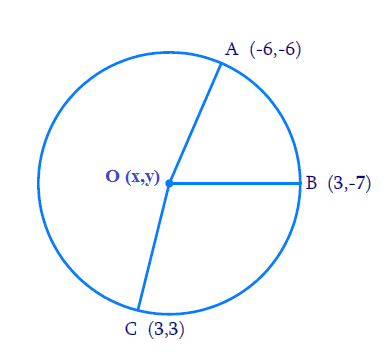Given,

• Let \begin{align}O\left( {x,y} \right)\end{align} be the centre of the circle.
• Let the points \begin{align}\left( {6,\, - 6} \right),\,\,\left( {3, - 7} \right),\,\,\text{and}\left( {3,3} \right)\end{align} be representing the points $$A$$, $$B$$, and $$C$$ on the circumference of the circle.

Distance from centre $$O$$ to $$A$$, $$B$$, $$C$$ are found below using the Distance formula mentioned in the Reasoning.

\begin{align}∴ OA & = \sqrt {{{(x - 6)}^2} + {{(y + 6)}^2}} \\ OB &= \sqrt {{{(x - 3)}^2} + {{(y + 7)}^2}} \\ OC &= \sqrt {{{(x - 3)}^2} + {{(y - 3)}^2}} \end{align}

From the figure that

\begin{align}OA &= OB \\ ( \text{radii of} & \text{ the same circle} ) \end{align}

\begin{align} \sqrt {\! (x\! - \!6)^2\! +\! (y \! +\! 6)^2 } & \! = \! \sqrt {\! (x \! -\! 3)^2 \!\! + \! (y \! + \! 7)^2 } \\\begin{bmatrix} {x^2} + 36 - 12x +\\ {y^2} + 36 + 12y \end{bmatrix} &\!=\! \begin{bmatrix} {x^2} + 9 - 6x +\\ {y^2} + 49 + 14y \end{bmatrix} \\\\\text{(Squaring on } & \text{both sides)}\\\\- 6x - 2y + 14 &= 0 \\ 3x + y &= 7 \ldots \text{Equation} (1) \end{align}

Similarly,

\begin{align}OA & = OC \\ ( \text{radii of} & \, \text{ the same circle} ) \end{align}

\begin{align} \! \sqrt { \! (x \! - \! 6)^2 \! + \! (y \! +\! 6)^2 } & \! = \! \sqrt {\! (x \! - \! 3)^2 \!+\! (y\! - \! 3)^2 }\\\begin{bmatrix} {x^2} + 36 - 12x + \\{y^2} + 36 + 12y \end{bmatrix} &= \begin{bmatrix} {x^2} + 9 - 6x + \\{y^2} + 9 - 6y \end{bmatrix}\\\\\text{(Squaring on } &\text{both sides)}\\\\- 6x + 18y + 54 &= 0 \\ - 3x + 9y &= - 27 \ldots(2) \end{align}

On adding Equation $$(1)$$ and Equation $$(2)$$, we obtain

\begin{align}10y &= - 20\\y &= - 2\end{align}

From Equation $$(1)$$,we obtain

\begin{align}3x - 2{\text{ }} = {\text{ }}7\\3x = {\text{ }}9\\x = {\text{ }}3\end{align}

Therefore, the centre of the circle is $$(3, -2)$$.

## Chapter 7 Ex.7.4 Question 4

The two opposite vertices of a square are \begin{align}\left( { - 1,\,2} \right){\text{ }}{\text{ and}}\left( {3,\,2} \right).\end{align} Find the coordinates of the other two vertices.

### Solution

Reasoning:

What is known?

The $$x$$ and $$y$$ co-ordinates of the two opposite vertices of a square.

What is unknown?

The coordinates of the other two vertices.

Steps:

From the Figure,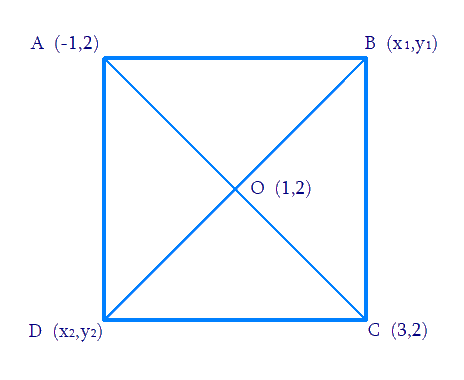Given,

• Let $$ABCD$$ be a square having known vertices \begin{align}{A}\,\,\left( { - 1,{\text{ }}2} \right){\text{ and}}{\text{ }}{C}\,\,\left( {3,{\text{ }}2} \right)\end{align}as vertices $$A$$ and $$C$$ respectively.
• Let \begin{align}\text{B}\left( {x, y} \right)\end{align} be one unknown vertex

We know that the sides of a square are equal to each other.

\begin{align}\therefore {{AB = BC}}\end{align}

By Using Distance formula to find distance between points $$AB\; {\rm and } \;BC ,$$

\begin{align} \sqrt {{{(x_1 \!-\! 1)}^2} \!+\! {{(y_1 \!-\! 2)}^2}} &\!=\! \sqrt {{{(x_1 \!-\! 3)}^2} \!+\! {{(y_1 \!-\! 2)}^2}} \\ \begin{bmatrix}{x_1^2} \!+\! 2x \!+\! 1 \!+\\ {y_1^2} \!-\! 4y \!+\! 4\end{bmatrix} &\!=\! \begin{bmatrix}{x_1^2} \!+\! 9 \!-\! 6x \!+\! \\{y_1^2} \!+\! 4 \!-\! 4y _1\end{bmatrix}\\\text{(By Simplifying }&\text{Transposing)}\\\\8x_1 &\!=\! 8 \\ x_1 &\!=\! 1 \end{align}

We know that in a square, all interior angles are of \begin{align}{90^\circ }.\end{align}

In \begin{align}\Delta ABC\end{align}

\begin{align}{AB}^{2}+{BC}^{2}={AC}^{2} \\\\\text { [By Pythagoras theorem }] \end {align}

Distance formula is used to find distance between $$AB$$, $$BC$$ and $$AC$$

\begin{align} &{\left( {\sqrt {{{(1 + 1)}^2} + {{(y_1 - 2)}^2}} } \right)^2} + {\left( {\sqrt {{{(1 - 3)}^2} + {{(y_1 - 2)}^2}} } \right)^2}\\ &\qquad= {\left( {\sqrt {{{(3 + 1)}^2} + {{(2 - 2)}^2}} } \right)^2} \end{align}

\begin{align}\begin{bmatrix}4 + {y_1^2} + 4 - 4y_1 + \\4 + {y_1^2} - 4y_1 + 4\end{bmatrix}&= 16 \\2{y_1^2} + 16 - 8y &= 16 \\2{y_1^2} - 8y &= 0\\y_1(y_1 - 4) &= 0\\y_1 &= 0\,\,or\,\,4\end{align}

Hence the required vertices are \begin{align}B{\text{ }}\left( {1,\,\,0} \right){\text{ }}{\text{ and}}\;D{\text{ }}\left( {1,{\text{ }}4} \right)\end{align}

## Chapter 7 Ex.7.4 Question 5

The class X students of a secondary school in Krishinagar have been allotted a rectangular plot of land for their gardening activity. Saplings of Gulmohar are planted on the boundary at a distance of $$1\; \rm m$$ from each other. There is a triangular grassy lawn in the plot as shown in the following figure. The students are to sow seeds of flowering plants on the remaining area of the plot.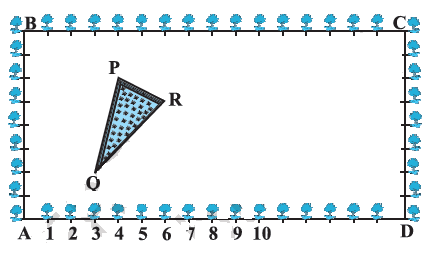(i) Taking $$A$$ as origin, find the coordinates of the vertices of the triangle.

(ii) What will be the coordinates of the vertices of $$\Delta$$ $$PQR$$ if $$C$$ is the origin? Also calculate the areas of the triangles in these cases. What do you observe?

### Solution

What is known?

Saplings of Gulmohar are planted on the boundary at a distance of $$1 \rm\,m$$ from each other.

What is unknown?

• The coordinates of the vertices of the triangle.
• The coordinates of the vertices of $$\Delta$$ $$PQR$$ if $$C$$ is the origin.

Steps:

(i) Given,

Taking $$A$$ as origin, we will take $$AD$$ as $$x$$-axis and $$AB$$ as $$y$$- axis.

It can be observed from the figure that the coordinates of point $$P$$, $$Q$$, and $$R$$ are $$(4, 6)$$, $$(3, 2)$$, and $$(6, 5)$$ respectively.

Let,
\begin{align}\text{P}\left( {{x_1},{y_1}} \right) = \left( {4,6} \right)\end{align}
Let,
\begin{align}\text{Q} \left( {{x_2},{y_2}} \right) = \left( {3,{\text{ }}2} \right)\end{align}
Let,
\begin{align}\text{R}\,\,\left( {{x_3},{y_3}} \right) = \left( {6,5} \right)\end{align}

Area of a triangle

\begin{align}\!=\begin{bmatrix}\!\frac{1}{2}{x}_{1}\left({y}_{2}\!-\!{y}_{3}\right)\!+\!{x}_{2}\left({y}_{3}\!-\!{y}_{1}\right)\!+\!\\{x}_{3}\left({y}_{1}\!-\!{y}_{2}\right)\end{bmatrix} \end{align}\, \ldots(1)

By substituting the values of vertices $$P$$, $$Q$$, $$R$$ in the Equation (1),

Area of triangle

\begin{align} PQR &= \frac{1}{2}\,\,\left[ {{{{x}}_1}\left( {{{{y}}_2} - {{{y}}_3}} \right) + {{{x}}_2}\left( {{{{y}}_3} - {{{y}}_1}} \right) + {{{x}}_3}\left( {{{{y}}_1} - {{{y}}_2}} \right)} \right]\\&= \frac{1}{2}\,\,[4(2 - 5) + 3(5 - 6) + 6(6 - 2)]\\&= \frac{1}{2}\,[ - 12 - 3 + 24]\\&= \frac{9}{2}\,\;\rm{}Square\;units\end{align}

(ii) Given,

• Taking $$C$$ as origin, $$CB$$ as $$x$$-axis, and $$CD$$ as $$y$$-axis
• The coordinates of vertices $$P$$, $$Q$$, and $$R$$ are $$(12, 2)$$, $$(13, 6)$$, and $$(10, 3)$$ respectively.
• Let \begin{align}\text{P}\left( {x_1,y1} \right) = \left( {12, 2} \right)\end{align}
• Let \begin{align}{\text{Q}}\left( {x_2,y2} \right) = \left( {13, 6} \right)\end{align}
• Let \begin{align}\text{R}\left( {x_3,y3} \right) = \left( {10,3} \right)\end{align}

Area of a triangle

\begin{align}\!=\!\begin{bmatrix}\frac{1}{2} \;{x}_{1}\left({y}_{2}\!-\!{y}_{3}\right)\!+\!{x}_{2}\left({y}_{3}\!-\!{y}_{1}\right)\!+\!\\{x}_{3}\left({y}_{1}\!-\!{y}_{2}\right)\end{bmatrix} \end{align} \ldots (1)

By substituting the values of vertices $$P$$, $$Q$$, $$R$$ in the Equation ($$1$$),

Area of triangle

\begin{align} PQR & \!=\! \frac{1}{2}\begin{bmatrix} {x_1}( {{y_2} \!-\! {y_3}} ) \!+\! {x_2}( {{y_3} \!-\! {y_1}} ) \!+\!\\ {x_3}( {{y_1} \!-\! {y_2} )} \end{bmatrix} \\&\!=\! \frac{1}{2}\begin{bmatrix} 12(6 \!- \!3) \!+\! 13(3 \!-\! 2)\!+\! \\ 10(2\! - 6) \end{bmatrix} \\ &\!= \!\frac{1}{2}\left[ {36 \!-\! 13 \!+\! 40} \right]\\ &\!=\! \frac{9}{2}\;\rm{}Square \;units \end{align}

It can be observed that the area of the triangle is same in both the cases.

## Chapter 7 Ex.7.4 Question 6

The vertices of a $$\Delta ABC$$ are $$A \;(4, 6)$$, $$B \;(1, 5)$$ and $$C\; (7, 2)$$. $$A$$ line is drawn to intersect sides $$AB$$ and $$AC$$ at $$D$$ and $$E$$ respectively, such that \begin{align}\frac{AD}{AB} = \frac{AE}{AC} = \frac{1}{4}\,\,.\end{align}

Calculate the area of the $$\Delta ADE$$ and compare it with the area of $$\Delta ABC$$.

(Recall Converse of basic proportionality theorem and Theorem 6.6 related to Ratio of areas of two similar triangles)

### Solution

Reasoning:

Converse of Basic Proportionality Theorem: If a line divides any two sides of a triangle in the same ratio, then the line must be parallel to the third side.

Theorem $$6.6$$ : The ratio of the areas of two similar triangles is equal to the square of the ratio of their corresponding sides.

What is known?

The $$x$$ and $$y$$ coordinates of the vertices of the triangle.

\begin{align}\frac{{AD}}{{AB}} = \frac{{AE}}{{AC}} = \frac{1}{4}\end{align}

where $$AB$$ and $$AC$$ are sides of the triangle intersected at $$D$$ and $$E$$.

What is unknown?

The area of the $$\Delta ADE$$ is to be calculated and compared with the area of $$\Delta ABC$$.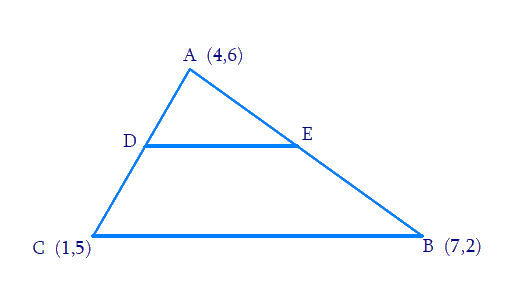Steps:

From the figure,

Given

\begin{align}\frac{{AD}}{{AB}} = \frac{{AE}}{{AC}} = \frac{1}{4}\end{align}

Therefore, $$D$$ and $$E$$ are two points on side $$AB$$ and $$AC$$ respectively such that they divide side $$AB$$ and $$AC$$ in a ratio of $$1:3$$.

By Section formula

\begin{align}{{P}}(x,y) \!\!= \!\!\left[ \frac{m x_2 \!+\! n x_1}{m \!+\! n},\frac{m y_2 \!+\! n y_1}{m \!+\! n} \right] \cdots (1) \end{align}

Coordintaes of Point D

\begin{align} &\!=\!\left[ {\frac{{1 \times 1 \!+\! 3 \times 4}}{{1 \!+\! 3}},\frac{{1 \times 5 \!+\! 3 \times 6}}{{1 \!+\! 3}}} \right] \\&\!=\! \left[ {\frac{{13}}{4},\frac{{23}}{4}} \right]{\text{ }}\\ \end{align}

Coordintaes of Point E

\begin{align} &\!=\! \left[ {\frac{{1 \times 7 \!+\! 3 \times 4}}{{1 \!+\! 3}},\frac{{1 \times 2 \!+\! 3 \times 6}}{{1 \!+\! 3}}} \right] \\ &\!=\! \left[ {\frac{{19}}{4},\frac{{20}}{4}} \right] \end{align}

Area of triangle

\begin{align}\!=\!\frac{1}{2} \begin{bmatrix} x_1 \left( y_2 \!-\! y_3 \right) + x_2 \left( y_3 \!-\! y_1 \right) +\\ x_3 \left( y_1 \!-\! y_2 \right) \end{bmatrix} \cdots (2) \end{align}

By substituting the vertices $$A$$, $$D$$, $$E$$ in $$(2)$$,

Area of $$\Delta ADE$$

\begin{align} &\!=\! \frac{1}{2} \begin{bmatrix} 4\left( {\frac{{23}}{4} \!-\! \frac{{20}}{4}} \right) \!+\! \frac{{13}}{4}\left( {\frac{{20}}{4} \!-\! 6} \right) \!+\!\\ \frac{{19}}{4}\left( {6 \!-\! \frac{{23}}{4}} \right) \end{bmatrix} \\&\!=\! \frac{1}{2}\left[ {3\! -\! \frac{{13}}{4} \!+\! \frac{{19}}{{16}}} \right] \\&\!=\! \frac{1}{2}\left[ {\frac{{48 \!-\! 52\! +\! 19}}{{16}}} \right] \\ &\!=\! \frac{{15}}{{32}}{\text{ Square units}}\end{align}

Clearly, the ratio between the areas of $$\Delta ADE$$ and $$\Delta ABC$$ is $$1:16$$.

Alternatively, we know that if a line segment in a triangle divides its two sides in the same ratio, then the line segment is parallel to the third side of the triangle[Converse of Basic Proportionality Theorem]. These two triangles so formed (here $$\Delta ADE$$ and $$\Delta ABC$$) will be similar to each other.

Hence, the ratio between the areas of these two triangles will be the square of the ratio between the sides of these two triangles.[Theorem 6.6]

Therefore, ratio between the areas of $$\Delta ADE$$ and

\begin{align}\Delta {\text{ABC}}\,{\text{ = }}\,{\left( {\frac{1}{2}} \right)^2} = \frac{1}{{16}}\end{align}

## Chapter 7 Ex.7.4 Question 7

Let $$A\;(4, 2)$$, $$B \;(6, 5)$$ and $$C \;(1, 4)$$ be the vertices of $$\Delta ABC$$.

(i) The median from $$A$$ meets $$BC$$ at $$D$$. Find the coordinates of point $$D$$.

(ii) Find the coordinates of the point $$P$$ on $$AD$$ such that $$AP: PD = 2:1$$

(iii) Find the coordinates of point $$Q$$ and $$R$$ on medians $$BE$$ and $$CF$$ respectively such that $$BQ: QE = 2:1$$ and $$CR: RF = 2:1$$.

(iv) What do you observe?

(v) If $$A(x_1, y_1)$$, $$B(x_2, y_2)$$, and $$C(x_3, y_3)$$ are the vertices of $$\Delta ABC$$, find the coordinates of the centroid of the triangle.

### Solution

Reasoning:

A median of a triangle is a line segment joining the vertex to the midpoint of the opposite side, thus bisecting that side. Every triangle has exactly three medians, one from each vertex, and they all intersect each other at the triangle's centroid.

What is known?

The $$x$$ and $$y$$ coordinates of the vertices of the triangle.

What is unknown?

The coordinates of point $$D$$, $$P$$, $$Q$$, $$R$$ and the co-ordinates of the centroid.

Steps:

From the figure,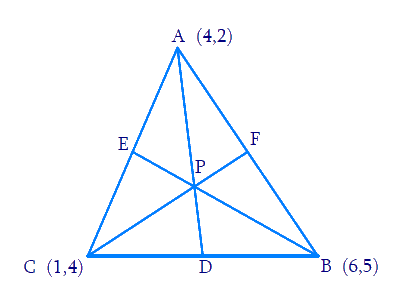Given,

• Let \begin{align}A \left( {{x_1}, {y_1}} \right) = \left( {4, 2} \right)\end{align}
• Let \begin{align}B \left( {{x_2}, {y_2}} \right) = \left( {6 , 5} \right)\end{align}
• Let \begin{align}C\left( {{x_3}, {y_3}} \right) = \left( {1, 4} \right)\end{align}

(i)

• Let $$AD$$ be the median of the triangle $$ABC$$
• Hence $$D$$ is the midpoint of $$BC$$ which can be found using the Mid Point formula.

\begin{align}M& = \left[ {\frac{{{x_1} + {x_2}}}{2},\frac{{{y_1} + {y_2}}}{2}} \right]\end{align}

Coordinates of $$D$$

\begin{align} &= \left[ {\frac{{6 + 1}}{2},\frac{{5 + 4}}{2}} \right] \\ &= \left[ {\frac{7}{2},\frac{9}{2}} \right]\end{align}

(ii) From the Figure,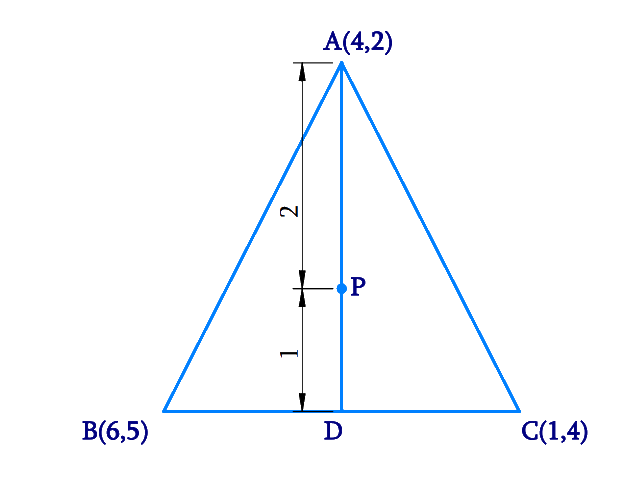Point $$P$$ divides the side $$AD$$ in a ratio $$m: n = 2: 1$$.

By Section Formula

\begin{align} P(x,y) & \!\!= \!\!\left[\!\! {\frac{{m{x_2} \!+\! n{x_1}}}{{m \!+\! n}},\frac{{m{y_2} \!+\! n{y_1}}}{{m \!+\! n}}} \!\! \right] \dots(1)\end{align}

Coordinates of $$P$$

\begin{align} & \!\!=\!\! \left[ {\frac{{2 \times \frac{7}{2} + 1 \times 4}}{{2 + 1}},\frac{{2 \times \frac{9}{2} + 1 \times 2}}{{2 + 1}}} \right] \\ &= \left[ {\frac{{11}}{3},\frac{{11}}{3}} \right]\end{align}

(iii) From the figure,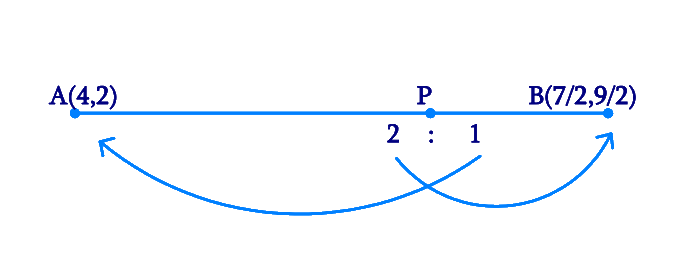Median $$BE$$ of the triangle will divide the side $$AC$$ in two equal parts.

Therefore, $$E$$ is the mid-point of side $$AC$$. The co-ordinates of $$E$$ can be calculated using the Mid-Point Formula.

\begin{align}M &= \left[ {\frac{{{x_1} + {x_2}}}{2},\frac{{{y_1} + {y_2}}}{2}} \right] \end{align}

Coordinates of $$E$$

\begin{align} &= \left[ {\frac{{4 + 1}}{2},\frac{{2 + 4}}{2}} \right] \\ &= \left[ {\frac{5}{2},3} \right]\end{align}

Point $$Q$$ divides the side $$BE$$ in a ratio $$2:1$$.

By Section Formula

\begin{align} P(x,y) & \!\!= \!\!\left[ {\frac{{m{x_2} \!+\! n{x_1}}}{{m \!+\! n}}\!,\!\frac{{m{y_2} \!+\! n{y_1}}}{{m \!+\! n}}} \right] \! \dots \!(1) \end{align}

Coordinates of $$Q$$

\begin{align} & \!\!= \!\!\left[ {\frac{{2 \times \frac{5}{2} + 1 \times 6}}{{2 + 1}},\frac{{2 \times 3 + 1 \times 5}}{{2 + 1}}} \right] \\ & = \left[ {\frac{{11}}{3},\frac{{11}}{3}} \right]\end{align}

Median $$CF$$ of the triangle will divide the side $$AB$$ in two equal parts.

Therefore, $$F$$ is the mid-point of side $$AB$$. The co-ordinates of $$F$$ can be calculated using the Mid-Point Formula.

Coordinates of $$F$$

\begin{align} &= \left[ {\frac{{4 + 6}}{2},\frac{{2 + 5}}{2}} \right] \\&= \left[ {5,\frac{7}{2}} \right]\end{align}

Point $$R$$ divides the side $$CF$$ in a ratio $$2: 1$$

By Section Formula

\begin{align} P(x,y) & \!\!= \!\!\left[ {\frac{{m{x_2}\! +\! n{x_1}}}{{m\! +\! n}}\!,\!\frac{{m{y_2} \!+\! n{y_1}}}{{m \!+\! n}}} \right] \!\dots \!(1) \end{align}

Coordinates of R

\begin{align} & \!\!= \!\!\left[ {\frac{{2 \times 5 + 1 \times 1}}{{2 + 1}},\frac{{2 \times \frac{7}{2} + 1 \times 4}}{{2 + 1}}} \right] \\ &= \left[ {\frac{{11}}{3},\frac{{11}}{3}} \right]\end{align}

(iv) It can be observed that the coordinates of point P, Q, R are the same. Therefore, all these are representing the same point on the plane i.e., the centroid of the triangle.

(v) Consider a triangle, $$\Delta ABC$$, having its vertices as

\begin{align}& A\left( {{x_1},\,{y_1}} \right),{\text{ }}B\left( {{x_2},\,{y_2}} \right),\end{align} and \begin{align}C\,\left( {{x_3},{y_3}} \right)\end{align}

Median $$AD$$ of the triangle will divide the side $$BC$$ in two equal parts.

Therefore, $$D$$ is the mid-point of side $$BC$$. The co-ordinates of $$D$$ can be calculated using the Mid-Point Formula.

Coordinates of  $$D$$

\begin{align} \!=\! \left[ {\frac{{{{x}_2} \!+\! {x_3}}}{2},\frac{{{{y}_2} \!+\! {y_3}}}{2}} \right]\end{align}

Let the centroid of this triangle be $$O$$.

Point $$O$$ divides the side $$AD$$ in a ratio $$2:1$$.

Coordinates of $$O$$

\begin{align} &\!=\! \left[ \frac{{2 \!\times\! \frac{{{x_2} +\! {x_3}}}{2} \!+\! 1 \!\times\! {x_1}}}{{2 \!+\! 1}}, \frac{{2 \!\times\! \frac{{{y_2} +\! {y_3}}}{2} \!+\! 1 \!\times\! {y_1}}}{{2 \!+\! 1}} \right] \\ &\!=\! \left[ {\frac{{{x_1} \!+\! {x_2} \!+\! {x_3}}}{3},\frac{{{y_1} \!+\! {y_2} \!+\! {y_3}}}{3}} \right]\end{align}

## Chapter 7 Ex.7.4 Question 8

$$ABCD$$ is a rectangle formed by the points $$A \;(- 1, - 1)$$, $$B\; (- 1, 4)$$, $$C \;(5, 4)$$ and $$D\; (5, - 1)$$. $$P$$, $$Q$$, $$R$$ and $$S$$ are the mid-points of $$AB$$, $$BC$$, $$CD$$, and $$DA$$ respectively. Is the quadrilateral $$PQRS$$ is a square? A rectangle? Or a rhombus? Justify your answer.

### Solution

Reasoning:

The distance between the two points can be measured using the Distance Formula which is given by:

Distance Formula

\begin{align}= \sqrt {{{\left( {{x_1} - {x_2}} \right)}^2} + {{\left( {{y_1} - {y_2}} \right)}^2}} .\end{align}

What is known?

The $$x$$ and $$y$$ coordinates of the vertices of the rectangle.

What is unknown?

To find whether quadrilateral $$PQRS$$ is a square? A rectangle? Or a rhombus?

Steps:

From the figure below,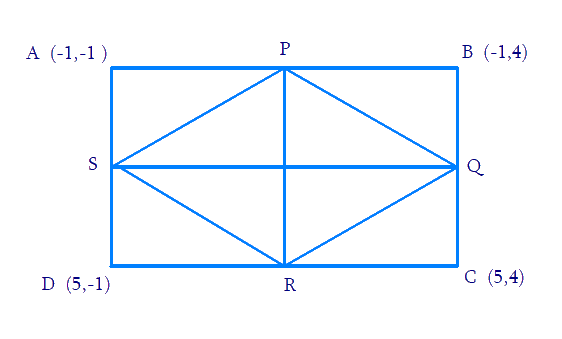$$P$$ is the mid-point of side $$AB$$. The co-ordinates of $$P$$ can be calculated using the Mid-Point Formula as

\begin{align}M = \left( {\frac{{{x_1} + {x_2}}}{2},\frac{{{y_1} + {y_2}}}{2}} \right)\end{align}

Therefore, the coordinates of $$P$$ are

\begin{align}\left[ {\frac{{ - 1 - 1}}{2},\,\frac{{ - 1 + 4}}{2}} \right) = \left( { - 1,\,\,\frac{3}{2}} \right] \end{align}

Similarly, the coordinates of $$Q$$, $$R$$ and $$S$$ are calculated using the Mid-Point Formula as :

$$(2,4),\left( {5,\frac{3}{2}} \right)\,{\text{and}}\,(2, - 1)$$ respectively.

We know that the distance between the two points is given by the Distance Formula,

\begin{align} & \sqrt {{{\left( {{x_1} - {x_2}} \right)}^2} + {{\left( {{y_1} - {y_2}} \right)}^2}} \ldots (1) \end{align}

Distance between two points $$P$$ and $$Q$$,

Length of $$PQ$$

\begin{align} &= \sqrt {{{( - 1 - 2)}^2} + {{\left( {\frac{3}{2} - 4} \right)}^2}} \\ &= \sqrt {9 + \frac{{25}}{4}} \\& = \sqrt {\frac{{61}}{4}} \end{align}

Distance between two points $$Q$$ and $$R$$,

Length of $$QR$$

\begin{align} &= \sqrt {{{(2 - 5)}^2} + {{\left( {4 - \frac{3}{2}} \right)}^2}} \\ &= \sqrt {9 + \frac{{25}}{4}} \\ &= \sqrt {\frac{{61}}{4}} \end{align}

Distance between two points $$R$$ and $$S$$,

Length of $$RS$$

\begin{align} &= \sqrt {{{(5 - 2)}^2} + {{\left( {\frac{3}{2} + 1} \right)}^2}} \\ &= \sqrt {9 + \frac{{25}}{4}} \\ &= \sqrt {\frac{{61}}{4}} \end{align}

Distance between two points $$S$$ and $$P$$,

Length of $$SP$$

\begin{align} &= \sqrt {{{(2 + 1)}^2} + {{\left( { - 1 - \frac{3}{2}} \right)}^2}} \\& = \sqrt {9 + \frac{{25}}{4}} \\ &= \sqrt {\frac{{61}}{4}}\end{align}

Distance between two points $$P$$ and $$R$$ which form the diagonal are ,

Length of $$PR$$

\begin{align} &= \sqrt {{{( - 1 - 5)}^2} + \left( {\frac{3}{2}} \right) - \frac{{{3^2}}}{2}} \\ &= 6\end{align}

Distance between two points $$Q$$ and $$S$$ which form the diagonal is calculated using the Distance Formula as,

Length of $$QS$$

\begin{align}{\text{ }}&= \,\sqrt {{{(2 - 2)}^2} + {{(4 + 1)}^2}} \\ &= 5\end{align}

It can be observed that all sides of the given quadrilateral are of the same measure. However, the diagonals are of different lengths. Therefore, $$PQRS$$ is a rhombus.

Download SOLVED Practice Questions of NCERT Solutions For Class 10 Maths Chapter 7 Exercise 7.4 for FREE
Ncert Class 10 Exercise 7.4
Ncert Solutions For Class 10 Maths Chapter 7 Exercise 7.4
Instant doubt clearing with Cuemath Advanced Math Program